# 这些高阶的函数技术，你掌握了么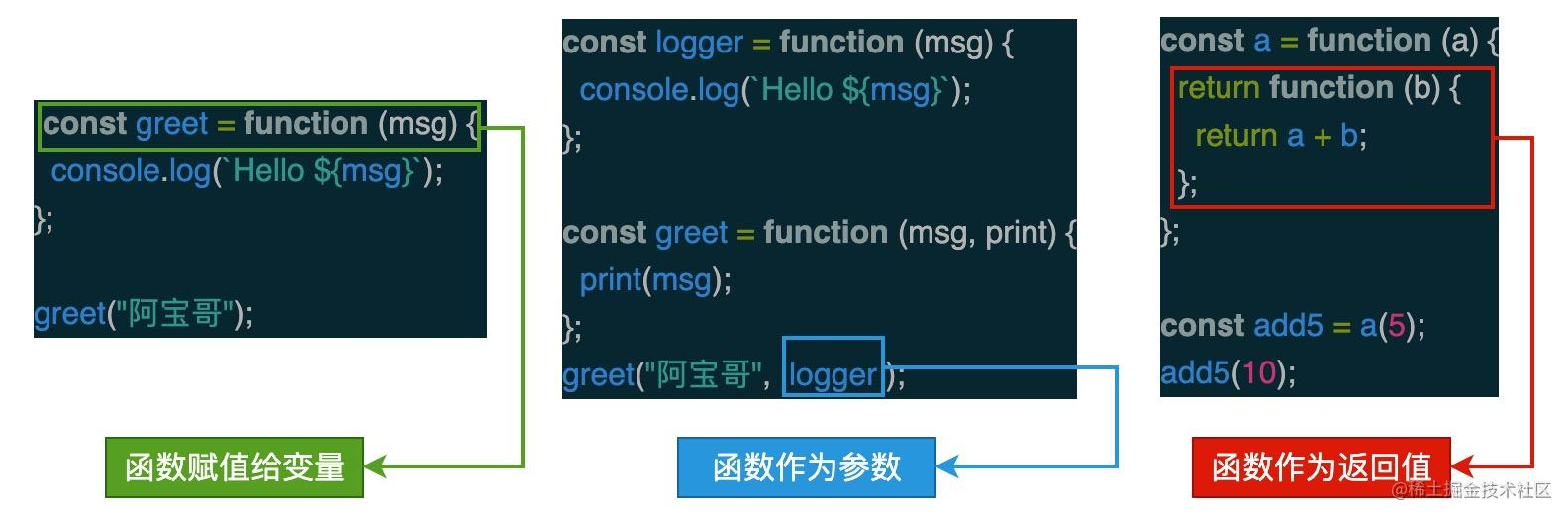### 一、高阶函数

• 接受一个或多个函数作为输入；
• 输出一个函数。

``````// Array.prototype.map 高阶函数
const array = [1, 2, 3, 4];
const map = array.map(x => x * 2); // [2, 4, 6, 8]

// Array.prototype.filter 高阶函数
const words = ['semlinker', 'kakuqo', 'lolo', 'abao'];
const result = words.filter(word => word.length > 5); // ["semlinker", "kakuqo"]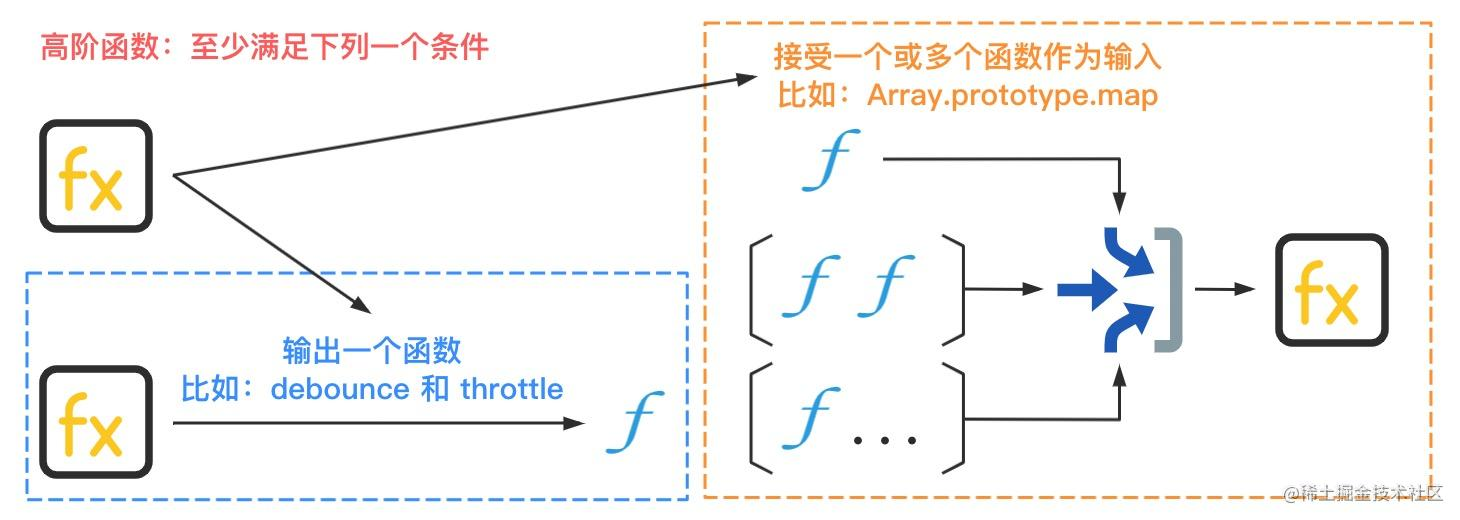### 二、函数组合

``````const composeFn = function (f, g) {
return function (x) {
return f(g(x));
};
};

#### 2.1 函数组合的作用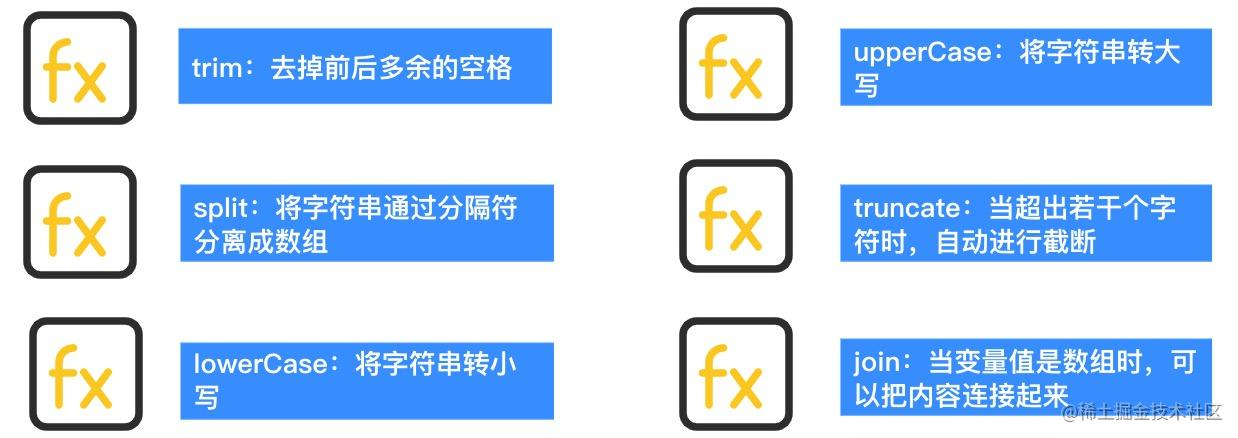``````function lowerCase(input) {
return input && typeof input === "string" ? input.toLowerCase() : input;
}

function upperCase(input) {
return input && typeof input === "string" ? input.toUpperCase() : input;
}

function trim(input) {
return typeof input === "string" ? input.trim() : input;
}

function split(input, delimiter = ",") {
return typeof input === "string" ? input.split(delimiter) : input;
}

// compose函数的实现，请参考 “组合函数的实现” 部分。
const trimLowerCaseAndSplit = compose(trim, lowerCase, split);
trimLowerCaseAndSplit(" a,B,C "); // ["a", "b", "c"]

#### 2.2 组合函数的实现

``````function compose(...funcs) {
return function (x) {
return funcs.reduce(function (arg, fn) {
return fn(arg);
}, x);
};
}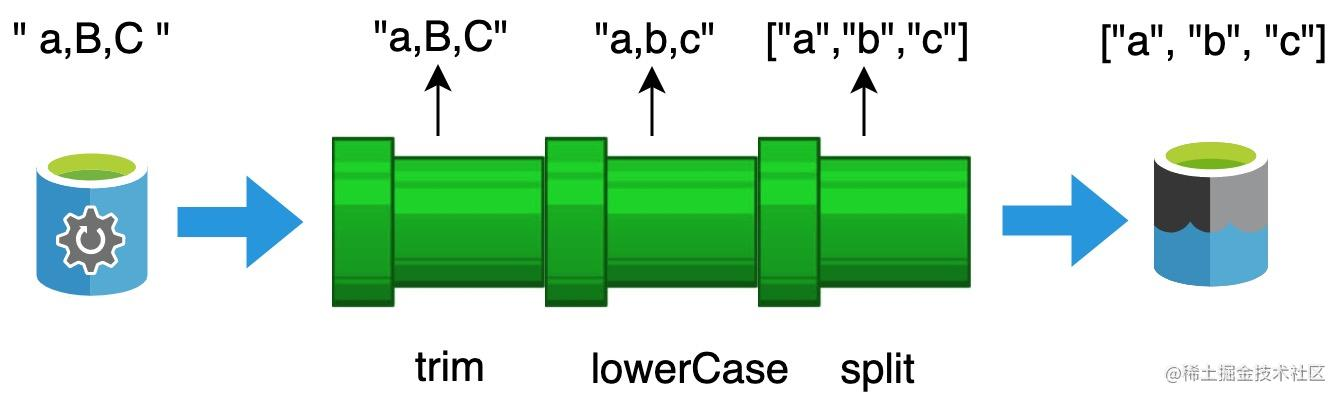``````function compose(middleware) {
// 省略部分代码
return function (context, next) {
let index = -1;
return dispatch(0);
function dispatch(i) {
if (i <= index)
return Promise.reject(new Error("next() called multiple times"));
index = i;
let fn = middleware[i];
if (i === middleware.length) fn = next;
if (!fn) return Promise.resolve();
try {
return Promise.resolve(fn(context, dispatch.bind(null, i + 1)));
} catch (err) {
return Promise.reject(err);
}
}
};
}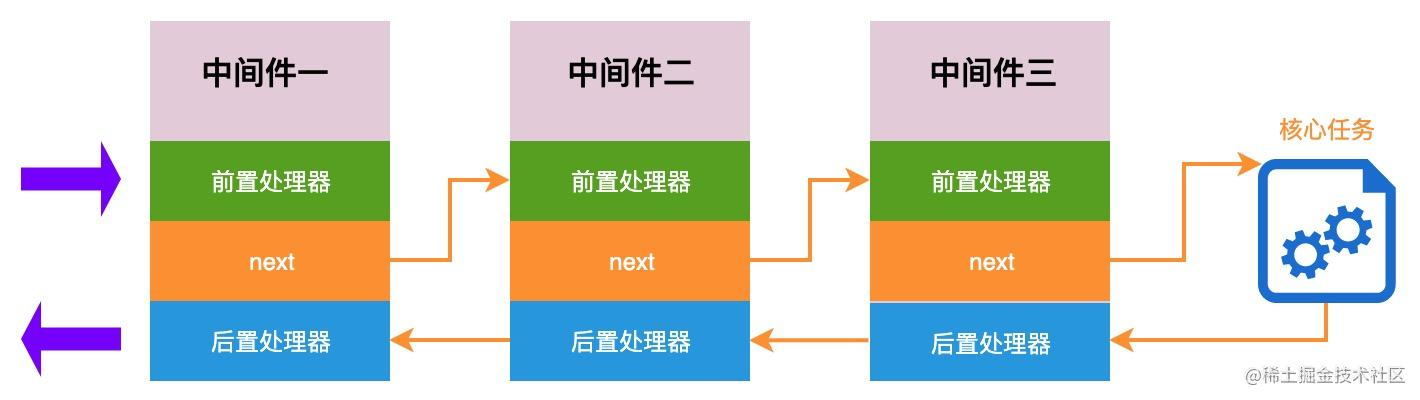### 三、柯里化

``````const func = function(a) {
return function(b) {
return a * a + b * b;
}
}

func(3)(4); // 25

Uncurrying 不是本文的重点，接下来我们使用 Lodash 提供的 `curry` 函数来直观感受一下，对函数进行 “柯里化” 处理之后产生的变化：

``````const abc = function(a, b, c) {
return [a, b, c];
};

const curried = _.curry(abc);

curried(1)(2)(3); // => [1, 2, 3]
curried(1, 2)(3); // => [1, 2, 3]
curried(1, 2, 3); // => [1, 2, 3]

_.curry(func, [arity=func.length])

#### 3.1 柯里化的作用

##### 3.1.1 参数复用
``````function buildUri(scheme, domain, path) {
return `\${scheme}://\${domain}/\${path}`;
}

const awesomeTsPath = buildUri("https", "github.com", "semlinker/awesome-typescript");

``````const _ = require("lodash");

const buildUriCurry = _.curry(buildUri);
const myGithubPath = buildUriCurry("https", "github.com");

##### 3.1.2 延迟计算/运行
``````const add = function (a, b) {
return a + b;
};

const plusOne = curried(1);

#### 3.2 柯里化的实现

``````function curry(func) {
return function curried(...args) {
if (args.length >= func.length) { // 通过函数的length属性，来获取函数的形参个数
return func.apply(this, args);
} else {
return function (...args2) {
return curried.apply(this, args.concat(args2));
};
}
}
}

### 四、偏函数应用

• 偏函数应用是固定一个函数的一个或多个参数，并返回一个可以接收剩余参数的函数；
• 柯里化是将函数转化为多个嵌套的一元函数，也就是每个函数只接收一个参数。

#### 4.1 偏函数的使用

``````function buildUri(scheme, domain, path) {
return `\${scheme}://\${domain}/\${path}`;
}

const myGithubPath = _.partial(buildUri, "https", "github.com");

_.partial(func, [partials])

#### 4.2 偏函数的实现

``````function partial(fn) {
let args = [].slice.call(arguments, 1);
return function () {
const newArgs = args.concat([].slice.call(arguments));
return fn.apply(this, newArgs);
};
}

#### 4.3 偏函数实现 vs 柯里化实现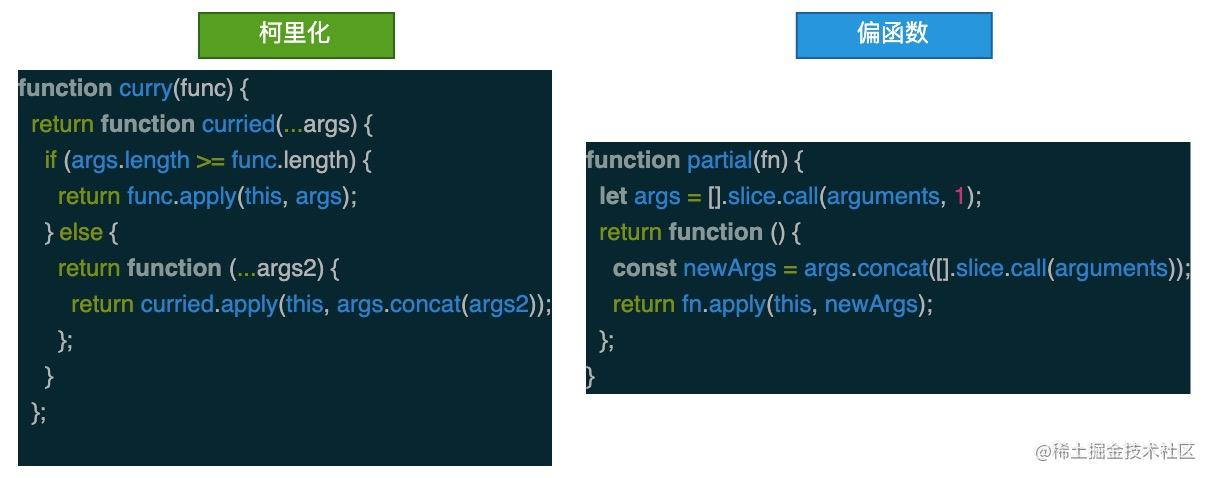### 五、惰性函数

``````function addHandler(element, type, handler) {
} else if (element.attachEvent) {
element.attachEvent("on" + type, handler);
} else {
element["on" + type] = handler;
}
}

#### 5.1 惰性载入函数

``````function addHandler(element, type, handler) {
addHandler = function (element, type, handler) {
};
} else if (element.attachEvent) {
addHandler = function (element, type, handler) {
element.attachEvent("on" + type, handler);
};
} else {
addHandler = function (element, type, handler) {
element["on" + type] = handler;
};
}
// 保证首次调用能正常执行监听
}

``````const addHandler = (function () {
return function (element, type, handler) {
};
} else if (document.attachEvent) {
return function (element, type, handler) {
element.attachEvent("on" + type, handler);
};
} else {
return function (element, type, handler) {
element["on" + type] = handler;
};
}
})();

### 六、缓存函数

``````function memorize(fn) {
const cache = Object.create(null); // 存储缓存数据的对象
return function (...args) {
const _args = JSON.stringify(args);
return cache[_args] || (cache[_args] = fn.apply(fn, args));
};
};

``````let complexCalc = (a, b) => {
// 执行复杂的计算
};

let memoCalc = memorize(complexCalc);
memoCalc(666, 888);
memoCalc(666, 888); // 从缓存中获取